# SWITCH Function

Evaluates a given expression (or a value) against a list of values and will return a result corresponding to the first matching value

## What is the SWITCH Function?

The SWITCH function is categorized under Excel’s Logical functions. This function will evaluate a given expression (or a value) against a list of values and will return a result corresponding to the first matching value. In case there is no matching value, an optional default value will be returned.

SWITCH was added in MS Excel 2016 and is unavailable in earlier versions. However, the function was previously available in VBA. The SWITCH function can be used instead of nested IF functions.

### Formula

=SWITCH(expression, value1, result1, [default or value2, result2],…[default or value3, result3])

The SWITCH function uses the following arguments:

1. Expression (required argument) – It can be a number, date, or some text that the function would compare. A few examples of the expression argument are as follows:

Type of ExpressionExample
Cell referenceA2
Logical testA2=“PASS”
Number or text2 or “FAIL”
Named rangeNamed_Range
Boolean valuesTRUE or FALSE
Math expressionA2+1

1. Value1 ………value126 (required argument) – This is the value that will be compared against the expression.
2. Result1 ………result126 – The value that will be returned when the corresponding valueN argument matches the expression.
3. Default (optional argument) – This is the value that the function should return if no matches are found in the valueN expression.

### How to use the SWITCH Function in Excel?

Let’s see a few examples to understand how the SWITCH function works:

#### Example 1

Let’s assume we are given a series of acronyms and we wish to return the value that the acronyms stand for.

The formula will be =SWITCH(A6,”DR”,”Debtors”,”CR”,”Creditors”,”JE”,”Journal Entry”,”LB”,”Ledger book”,”unknown”).

We get the result below: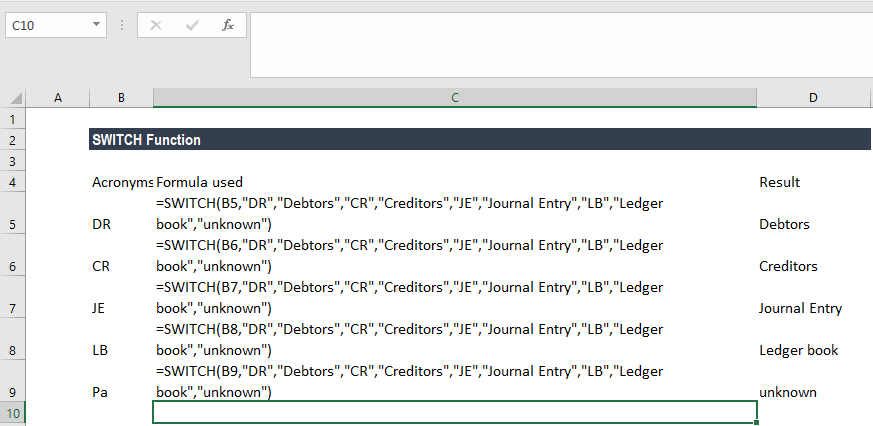If we need to use an IF nested formula, it would be a long formula. Both SWITCH function and IF function help us to specify a series of conditions. However, with SWITCH, we can define an expression and a sequence of values and results, not several conditional statements.

The best part of the SWITCH function is that we don’t need to repeat the expression several times, which sometimes happens in nested IF formulas.

Now if we needed to use the IF function, the formula would’ve been:

=IF(B5=”DR”,”Debtors”,IF(A2=”CR”,”Creditors”,IF(B5=”JE”,”Journal Entry”,IF(B5=”LB”,”Ledger Book”,”unknown”))))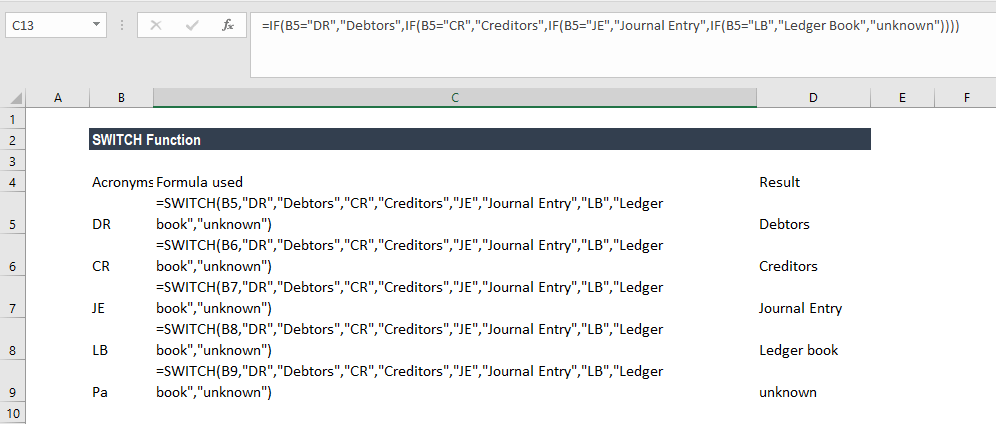#### Example 2 – Using SWITCH with other functions

Suppose we are given a few dates and we want to see if they refer to present day, tomorrow, or yesterday. Using the SWITCH, DAYS, and TODAY functions together, we can achieve the desired results.

The TODAY function will return the serial number of the current date, and the DAYS function will return the number of days between two dates.

The formula to use is =SWITCH(DAYS(TODAY(),B5), 0, “Present date”, 1,”Yesterday”, -1,”Tomorrow”,”Unknown”).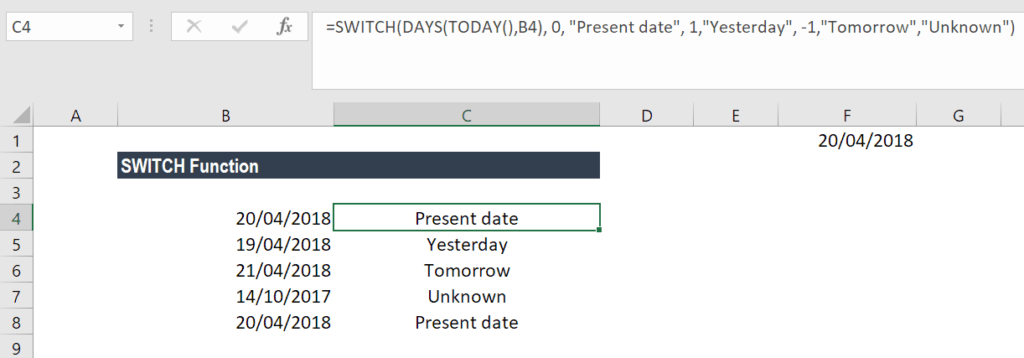#### Example 3

In some countries, the financial year starts on a date other than January 1, so they often need to classify dates into fiscal quarters. For example, if April to March is the financial year, then April-June would be quarter 1 and so on. We can use SWITCH in such scenarios: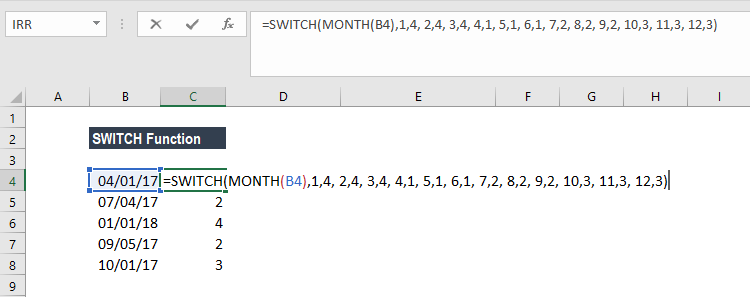We get the results below: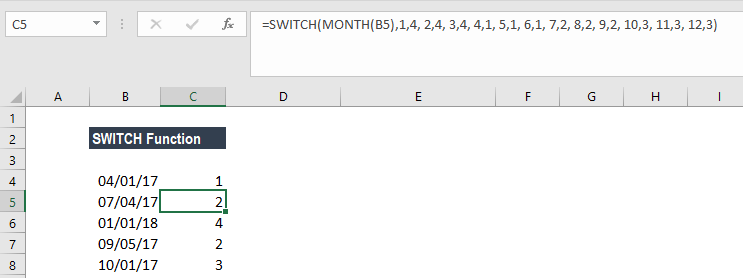We used a formula here that would find the month number in the Dates column: if it’s month 1, it’s quarter 4; if it’s month 2, it’s quarter 4; if it’s month 3, it’s quarter 4; if it’s month 4, it’s quarter 1; and so on.

### A few notes about the SWITCH Function

1. As the functions are limited to 254 arguments, we can use up to 126 pairs of value and result arguments.
2. #N/A error – Occurs when the SWITCH function is unable to match and there is no else argument. For example, for a value 7 and we give the formula =SWITCH(A3,1,”Sunday”,2,”Monday”,3,”Tuesday”), the function will return the #N/A error.
3. #NAME? error – Occurs if we edit a cell in a version of Excel that doesn’t include the SWITCH function.

Thanks for reading CFI’s guide to the Excel SWITCH function. By taking the time to learn and master Excel functions, you’ll significantly speed up your financial analysis. To learn more, check out these additional CFI resources:

• Excel Functions for Finance